###### back to index | new

What is the radius of a circle inscribed in a triangle with sides of length $5$, $12$ and $13$ units?

Triangle $ABC$ has a right angle at $B$. Point $D$ is the foot of the altitude from $B$, $AD=3$, and $DC=4$. What is the area of $\triangle ABC$?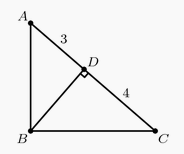A flagpole is originally $5$ meters tall. A hurricane snaps the flagpole at a point $x$ meters above the ground so that the upper part, still attached to the stump, touches the ground $1$ meter away from the base. What is $x$?

A right triangle has perimeter $32$ and area $20$. What is the length of its hypotenuse?

A triangle with side lengths in the ratio $3 : 4 : 5$ is inscribed in a circle with radius 3. What is the area of the triangle?

Given a triangle with side lengths 15, 20, and 25, find the triangle's smallest height.

The inradius of triangle $ABC$ is $1$ and the side lengths of $ABC$ are all integers. Prove that triangle $ABC$ is right-angled.

Square $ABCD$ has side length $2$. A semicircle with diameter $\overline{AB}$ is constructed inside the square, and the tangent to the semicircle from $C$ intersects side $\overline{AD}$ at $E$. What is the length of $\overline{CE}$?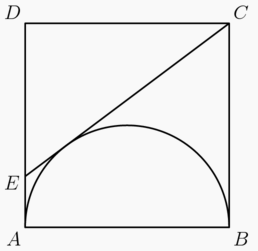Let $\triangle{ABC}$ be a Pythagorean triangle. If $\triangle{ABC}$'s circumstance is 30, find its circumcircle's area.

Let $\triangle{ABC}$ be a right triangle whose sides lengths are all integers. If $\triangle{ABC}$'s perimeter is 30, find its incircle's area.

Let $\triangle{ABC}$ be a right triangle where $\angle{C} = 90^\circ$. If point $D$ is on side $BC$ or its extension, show that $$AB^2 = DB^2 + DA^2 \pm 2 \cdot DB \cdot DC$$ If $D$ is on $BC$, then the $3^{rd}$ term above takes a positive coefficient. Otherwise, if $D$ is on its extension, it takes a negative coefficient.

An isosceles triangle with equal sides of 5 and bases of 6 is inscribed in a circle. Find the radius of that circle.

Triangle $ABC_0$ has a right angle at $C_0$. Its side lengths are pariwise relatively prime positive integers, and its perimeter is $p$. Let $C_1$ be the foot of the altitude to $\overline{AB}$, and for $n \geq 2$, let $C_n$ be the foot of the altitude to $\overline{C_{n-2}B}$ in $\triangle C_{n-2}C_{n-1}B$. The sum $\sum_{i=1}^\infty C_{n-2}C_{n-1} = 6p$. Find $p$.

Rectangle $ABCD$ is shown with $AB = 6$ units and $AD = 5$ units. If $AC$ is extended to point $E$ such that $AC$ is congruent to $CE$, what is the length of $DE$?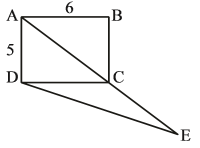Line $PT$ is tangent to circle $O$ at point $T$. $PA$ intersects circle $O$ and its diameter $CT$ at $B$, $D$, and $A$ in that order. If $CD=2, AD=3,$ and $BD=6$, find the length of $PB$.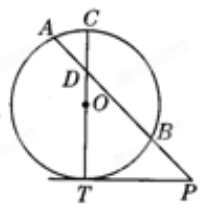(British Flag Theorem) Let point $P$ lie inside rectangle $ABCD$. Draw four squares using each of $AP$, $BP$, $CP$, and $DP$ as one side. Show that $$S_{AA_1A_2P}+S_{CC_1C_2P}=S_{BB_1B_2P}+S_{DD_1D_2P}$$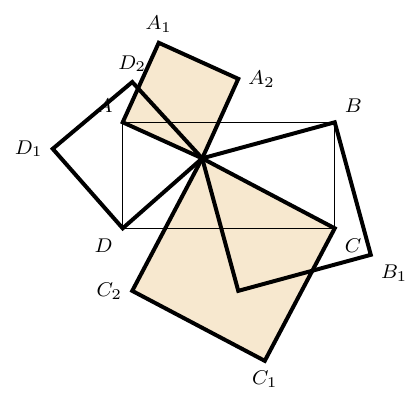(De Gua's Theorem) In a trirectangular tetrahedron $ABCD$ where $A$ is the shared right-angle corner. Show that $$S_{\triangle{BCD}}^2=S_{\triangle{ABC}}^2+S_{\triangle{ACD}}^2+S_{\triangle{ADB}}^2$$

Let $CD$ be the altitude in right $\triangle{ABC}$ from the right angle $C$. If inradii of $\triangle{ABC}$, $\triangle{ACD}$, and $\triangle{BCD}$ be $r_1$, $r_2$, and $r_3$, respectively, show that $$r_1 + r_2 + r_3 = CD$$

Three circles are tangent to each other and also a common line, as shown. Let the radii of circles $O_1$, $O_2$, and $O_3$ be $r_1$, $r_2$, and $r_3$, respectively. Show that $$\frac{1}{\sqrt{r_3}}=\frac{1}{\sqrt{r_1}} +\frac{1}{\sqrt{r_2}}$$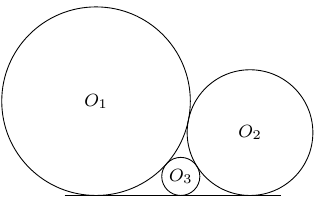Given two segments $AB$ and $MN$, show that $$MN\perp AB \Leftrightarrow AM^2 - BM^2 = AN^2 - BN^2$$

Let point $P$ inside an equilateral $\triangle{ABC}$ such that $AP=3$, $BP=4$, and $CP=5$. Find the side length of $\triangle{ABC}$.

Let $M$ be a point inside $\triangle{ABC}$. Draw $MA'\perp BC$, $MB'\perp CA$, and $MC'\perp AB$ such that $BA'=BC'$ and $CA'=CB'$. Prove $AB'=AC'$.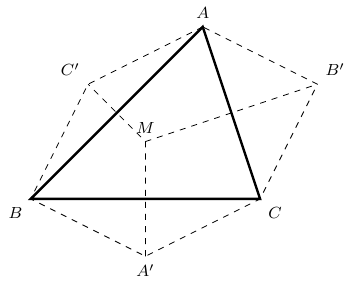Find one root to $\sqrt{3}x^7 + x^4 + 2=0$.